# MCQs on Electric Circuits

##### Page 57 of 63. Go to page 1 2 3 4 5 6 7 8 9 10 11 12 13 14 15 16 17 18 19 20 21 22 23 24 25 26 27 28 29 30 31 32 33 34 35 36 37 38 39 40 41 42 43 44 45 46 47 48 49 50 51 52 53 54 55 56 57 58 59 60 61 62 63
01․ A 3 – φ delta connected symmetrical load consumes P Watts of power from a balanced supply. If the same load is connected in star to the same supply then what is the power consumption?
P/3.
P.
√3P.
3P.

PΔ = 3PY

02․ Condition for a balanced 3 - phase load is that, each phase has the same value of __________________.
impedance.
resistance.
power factor.
all of these.

The condition of a 3 - phase balanced are that each phase has the same value of impedance, resistance and power factor.

03․ A 3 wire, 3 - φ supply feeds a load consisting of three equal resistors. By how much is the load reduced if one of the resistors be removed when the load is in star?
25 % of the original load.
50 % of the original load.
33.33 % of the original load.
66.66 % of the original load.

If one resistor is removed from a 3 - φ circuit, then the circuit is no longer 3 - φ but converted into single phase circuit, having two resistor, each of R Ω connected in series across the supply of VL Volts.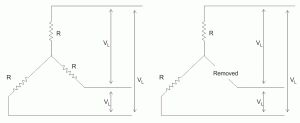04․ Phase voltages of a star connected alternator are ER = 240∠0o V, EY = 240∠-120o V and EB = 240∠ + 120o V. What is the phase sequence of the system?
RYB.
RBY.
YBR.
BYR.

Here ER is reference voltage EY lags behind it by 120o, EB lags behind ER by 240o and EY by 120o. So phase sequence is RYB.

05․ A balanced three Phase Y-connected load has one phase voltage VB = 277∠45o V. The phase sequence is RYB. The line to line voltage VRY is
480∠45o V.
480∠ - 45o V.
339∠45o V.
339∠- 45o V.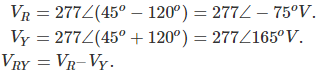06․ In delta connected circuit when one resistor is open, then power will be
0.
increased by factor of 3.
reduced by the factor of 3.
remain unchanged.

Power consumed in case of balanced delta connection P = Vph2/Req. Req = 2R/3. So, P = 3Vph2/2R. If one resistor is removed from a 3 - phase delta connected circuit, then the circuit will be converted into single phase circuit having two resistors in series. P’ = Vph2/Req. Req = 2R. So, P’ = Vph2/2R. P’ = P/3

07․ For balanced star load, if unbalanced line current are IR, IY, IB equal to - j10, - j10 and - j20 respectively, the neutral current will be equal to
(8.66 + j5) A.
(8.66 - j5) A.
(-8.66 - j5) A.
(-9.66 + j5) A.

All three current will be separated by 120o in space.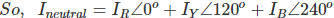08․ VRN, VYN and VBN are three phase voltage of a balanced three phase system. If VRN = 200∠30o V and VBN = 200∠- 90o V, then phase sequence of the system is
RYB.
RBY.
YRB.
BRY.

Phase Y lags phase R by (30 + 90)o = 120o so the phase sequence RBY.

09․ Three identical inductors are connected in delta with a 220 V, 50 Hz, 3 - φ, supply. If the phase current is 24 A, then what is the value of each of the inductance?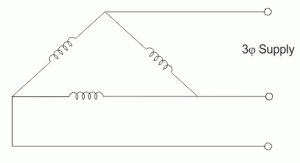0.058 H.
0.049 H.
0.029.
None of these.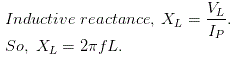10․ A balanced 3 - φ star connected load is fed from a 208 V, 3 - φ supply. Each load has resistance of 35 Ω. The total power is
411.3 W.
618 W.
1236 W.
none of these.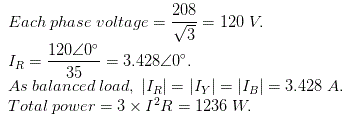<<<5556575859>>>